•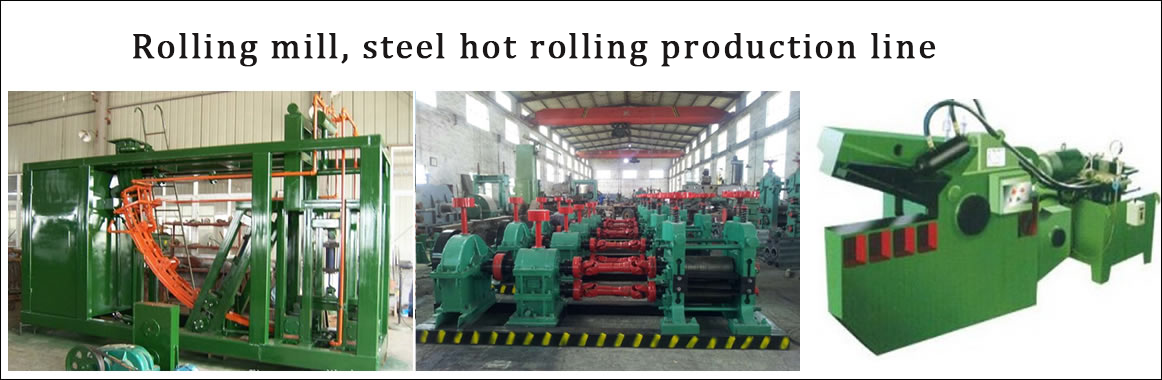•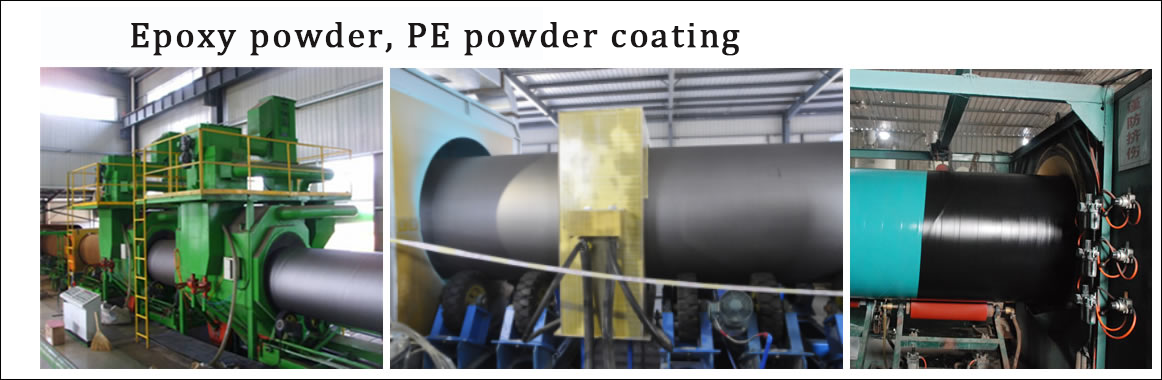•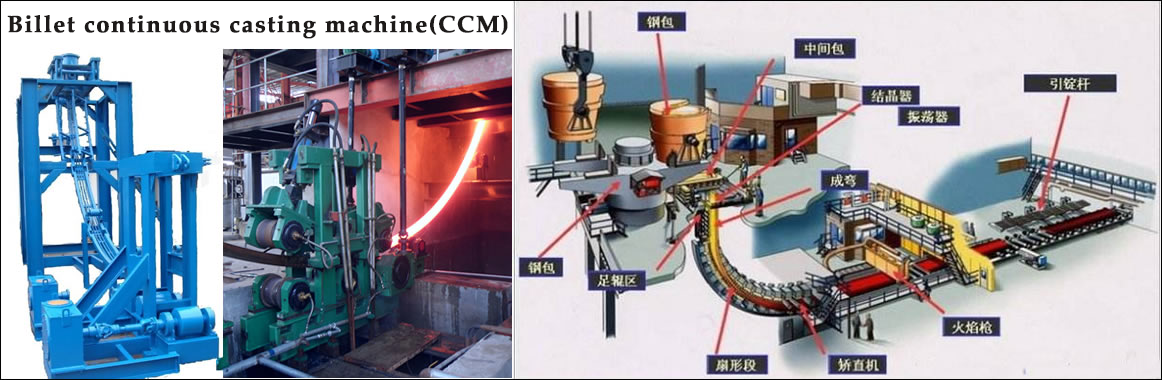•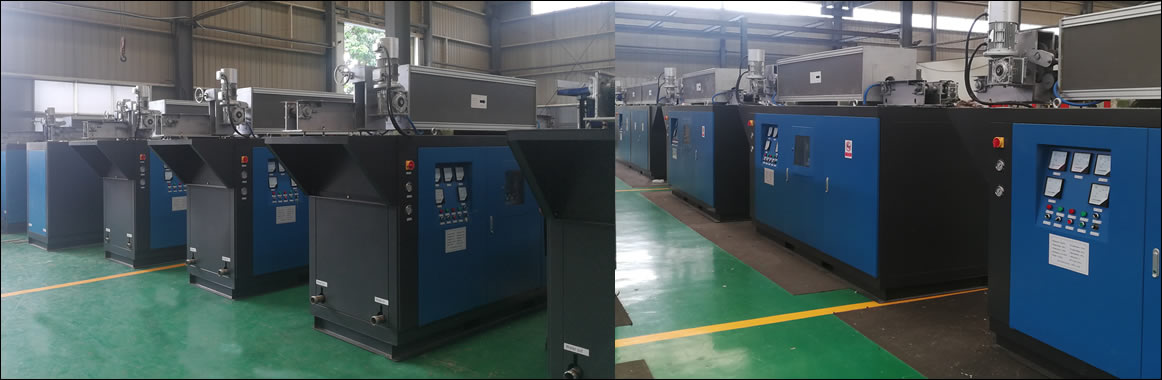•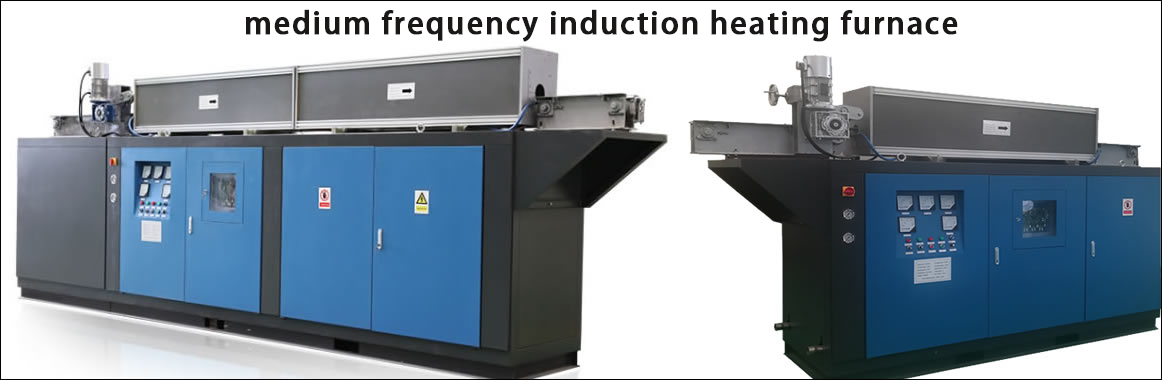•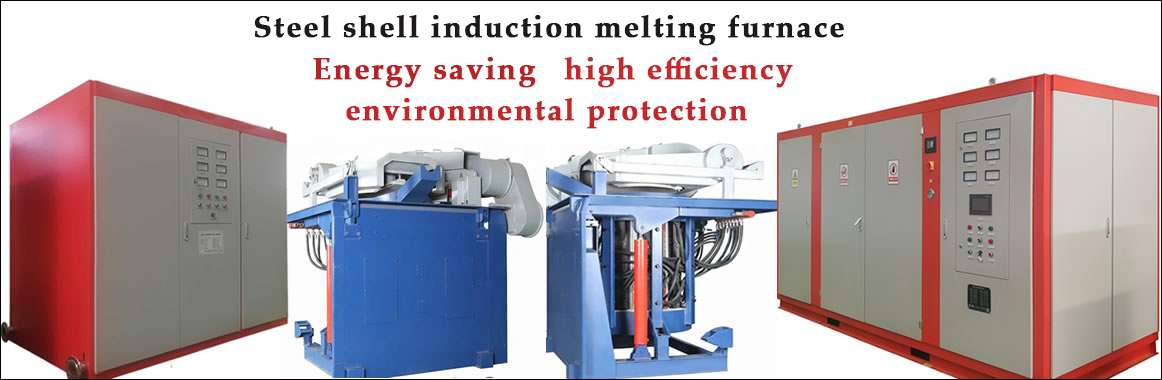Contact
Tel: 18037961302
Sales hot line ( 24 hours  service): 18037961302

E-Mail: firstfurnace@gmail.com

whatsapp:+8618037961302

Adress: Luoxin Industrial Park, Luoyang, Henan
Electric furnace technical parameters

What is the meaning of the intermediate frequency furnace power factor?

The most basic explanation of the power factor of the intermediate frequency furnace: the power of the intermediate frequency furnace is 100 units, that is, 100 units of power is delivered to the intermediate frequency furnace. However, due to the inherent reactive losses of most electrical systems, only 70 units of power can be used. Unfortunately, although only 70 units are used, there is a fee of 100 units. In this example, the power factor is 0.7 (if most of the intermediate frequency furnace's power factor is less than 0.9, it will be fine), this reactive power is mainly found in the motor's intermediate frequency furnace (such as blowers, pumps, compressors, etc.). Also called inductive load. Power factor is a measure of motor performance.

Basic explanation of the power factor of the intermediate frequency furnace: Each motor system consumes two large powers, which are real useful work (called kilowatts) and reactive useless work. The power factor is the ratio of useful work to total power. The higher the power factor, the higher the ratio of useful work to total power, and the more efficient the system is.

High-frequency explanation of the intermediate frequency furnace power factor: In the inductive load circuit, the peak value of the current waveform occurs after the peak of the voltage waveform. The separation of the two waveform peaks can be expressed in terms of power factor. The lower the power factor, the larger the separation of the two waveform peaks. Paulkin can bring the two peaks back together, which increases the efficiency of the system.

Power factor is one of the important technical data of AC circuits. The level of power factor is of great significance for the utilization and analysis of electrical intermediate frequency furnaces and the study of power consumption. The so-called power factor refers to the cosine of the difference between the voltage U between the two ends of the network (the circuit with two contacts to the outside world) and the current I. The power consumed in the two-end network refers to the average power, also called active power, which is equal to: P = UIcos Φ, from which it can be seen that the power P consumed in the circuit depends not only on the magnitude of the voltage V and the current I. Also related to power factor. The magnitude of the power factor depends on the nature of the load in the circuit. For resistive loads, the phase difference between voltage and current is 0, therefore, the power factor of the circuit is the largest (); and for purely inductive circuits, the phase difference between voltage and current is π/2, and it is the voltage lead current; in pure capacitance In the circuit, the phase difference between voltage and current is -(π/2), that is, the current leads the voltage. In the latter two circuits, the power factor is zero. For a general load circuit, the power factor is between 0 and 1.

Generally speaking, in the two-end network, improving the power factor of the electrical appliance has two meanings. First, it can reduce the power loss on the transmission line; second, it can fully utilize the electric power intermediate frequency furnace (such as generators, transformers, etc.). potential. Because the appliance always works under certain conditions U and a certain active power P, by the formula I=P/UcosΦ

It can be seen that if the power factor is too low, it is necessary to use a larger current to ensure the normal operation of the electrical appliance, and at the same time, the transmission current on the transmission line increases, resulting in an increase in Joule heat loss on the line. In addition, the voltage drop across the resistance of the transmission line and the inner group of the power supply is proportional to the current in the consumer, and increasing the current necessarily increases the voltage loss inside the transmission line and the power supply. Therefore, by increasing the power factor of the electrical appliance, the transmission current can be reduced, thereby reducing the power loss on the transmission line.

It is not difficult to understand that increasing the power factor and increasing the power factor can give full play to the potential of the power intermediate frequency furnace. Because any electric intermediate frequency furnace is always working within a certain rated voltage and rated current limit. If the working voltage exceeds the rated value, it will threaten the insulation performance of the intermediate frequency furnace; if the working current exceeds the rated value, the internal temperature of the intermediate frequency furnace will rise too high, thus reducing the service life of the intermediate frequency furnace. For the electric power intermediate frequency furnace, the product of the voltage and current rating is called the rated apparent power S of the intermediate frequency furnace, that is, the S amount = U amount I, which is also called the capacity of the intermediate frequency furnace. For the generator, This capacity is the maximum power that the generator can output. It marks the power generation potential of the generator. As for the actual power output of the generator, it is related to the power factor of the appliance. The power consumed by the appliance is P=S amount cosΦ.

The high power factor means that the active power accounts for a large proportion of the rated apparent power, and the electric energy output by the generator is fully utilized. For example, if the capacity of the generator is 15,000 kVA, when the power factor of the power system is increased from 0.6 to 0.8, the actual power generation capacity of the generator can be increased by 3000 kW, which is not the function of the generator. Potential? The use of intermediate frequency furnaces is also more reasonable. From this perspective, the power factor can be expressed as the ratio of active power to machine power, ie cosΦ = P / S amount. Now the power factor of the series one to two intermediate frequency furnace is greater than or equal to 0.95.